Peter Hilton, Jean Pedersen, Hans Walser

The Faces of the Tri-Hexaflexagon

Mathematics Magazine, Vol. 70, No. 4, October 1997, 243-251Introduction

Hexaflexagons were invented at Princeton in 1939 by Arthur H. Stone, then a graduate student, now Professor Emeritus of Mathematics at the University of Rochester. Martin Gardner gives an interesting account (see ) of Stone’s work and his collaboration with Bryant Tuckerman, then a graduate student and now a retired research mathematician from IBM (Yorktown Heights, NY), the late Richard P. Feynman, then a graduate student in physics and later a Nobel Laureate, and John W. Tukey, then a young mathematics instructor and now an Emeritus Professor at Princeton. It is interesting to remark that the diagrams Feynman devised for analyzing 6-faces hexaflexagons were forerunners of the famous Feynman Diagrams in modern atomic physics. A description of how to construct a 3-faces hexaflexagon may be found in any of the references  through . Further, a detailed description is given in [3, p. 63-74] of how to construct hexaflexagons with 3n faces without the use of straightedge or compass.

The particular hexaflexagons we will consider in this article is the tri-hexaflexagon, so named because it has 3 faces; that is, in any given state of the flexagon, one face (consisting of 6 equilateral triangles) will be up, one face will be down, and one face will be hidden. Although the orientation of the faces will vary from state to state, the same 6 triangles will always appear together on a face.

We will show in this article how, by drawing a human visage on each face of the flexagon, and using a different color for each face, we can keep track of all the possible positions of the flexagon as it lies in a plane. We are thereby able to discover that the set of motions of this flexagon which bring it into coincidence with itself constitutes the dihedral group.

1.         How to build the faced hexaflexagon

The hexaflexagon is constructed from a strip of paper containing 10 equilateral triangles as shown in FIGURE 1. In order that the final model will flex easily the fold lines between the triangles should be creased firmly in both directions.FIGURE 1: The strip

Now we decorate both sides of the strip as shown in FIGURE 2, where we make the bottom surface of the strip visible by flipping the entire pattern piece over a horizontal axis as indicated by the figure (where the vertices A, B, C, D should correspond with the vertices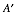,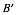,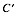,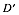, respectively, after you have flipped the piece over). Caution: Be careful here! Flipping the pattern piece over a vertical axis, and the decorating it as shown does not produce the desired flexagon.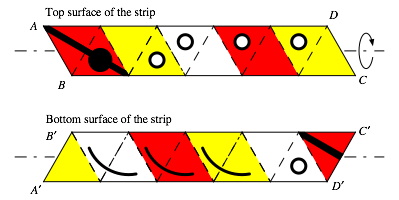FIGURE 2: Decorating the strip

Now we suggest that you view the construction of the flexagon as a puzzle. Here are some hints for constructing the flexagon with smiling (and frowning) faces.

(1)   The first triangle of the upper portion of the strip is ultimately glued to the last triangle on the bottom portion (and it doesn’t matter which one is on top of which). We suggest that you attach these triangles with a paper clip at first, and save the actual gluing until you are certain about the correctness of the construction.

(2)   The completed flexagon should show the visage of a smiling face, entirely in white, as you lay down as shown in FIGURE 3(a). And when you flip the flexagon over, about a horizontal axis, it should show the visage of a frowning face, entirely in yellow, oriented as displayed in FIGURE 3(b).

(3)   The strip that created the hexagon contains three half-twists; thus like the Möbius band, it has only one surface (or side). Geometrically this means there will be three slits on any face of this flexagon, symmetrically located at 120° intervals about its center. These slits are created by edges of the strip that go from alternate vertices of the hexagon to its center as shown in both parts of FIGURE 3.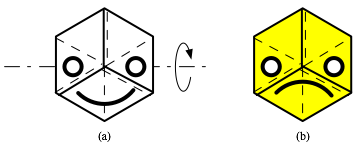FIGURE 3: The tri-hexaflexagon

From the last hint above we know that the flexagon now has only one surface. After you become proficient at manipulating your flexagon you may wish to verify with your own model that the repetitive pattern of the three mouths, three right eyes, and three left eyes in the colors white, red, yellow, respectively, occurs as shown in FIGURE 4.FIGURE 4: The entire surface of the strip

2.         The happy group

First we will always need to start with the flexagon in a standard initial position, that is, with the smiling white face up and oriented precisely as shown in FIGURE 5(a).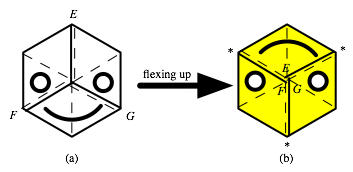FIGURE 5: The motion f, flexing up

Now we assumeand define the following motions:

the identity motion 1, which means we retain the initial position,

f = the motion of flexing up, starting from the initial position,

f n = the motion of flexing up n times, starting from the initial position.

More precisely, the motion f consists of lifting the vertices of the hexagon, labeled E, F, and G (in FIGURE 5(a)) above the flexagon until they meet, when the flexagon will come apart at the bottom and fall into the shape of a new hexagon with the vertices E, F, and G at its center. If this is done correctly (it is important not to rotate the flexagon in either direction), you wll see the upside-down smiling yellow face shown in FIGURE 5(b). Notice that the slits in the flexagon have revolved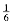of a turn. Thus, when you flex up the second time you will have to bring the vertices marked with asterisks (*) together above the flexagon. A simple way to remember what to do is that, in each case, the vertex at the forehead of the human visage gets lifted to the center (and it disappears as the motion is completed).

We now follow the usual, obvious procedure of identifying a motion with its effect on the initial position. When we do this we see that f 18 = 1, the identity motion.

Once you have mastered the motions f n, you may verify the sequence of motions which produce the Happy Group shown in FIGURE 6; here we have adopted the identification indicated above.

Since f 18 is the identity, we see that the Happy Group is the cyclic group C18, generated by f.

Next we define flexing down. To describe this motion, we begin, as before, with the flexagon in the standard initial position shown in FIGURE 7(a). Then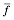means that we push the vertices of the hexagon labeled H, J, and K downwards until they meet; at that stage the flexagon will come apart at the top and fall into the shape of a new hexagon with the vertices H, J, and K at its center, but underneath the hexagon (this is indicated by putting H, J, and K in parentheses in FIGURE 7(b)). If this is done correctly, we will obtain the smiling pirate face in red as shown in FIGURE 7(b).FIGURE 6: The Happy Group

Just as with the up-motions, it is important not to rotate the flexagon in either direction as we flex it. Ta obtain, we simply repeat the process of flexing down n times (notice that when we flex down the second time it is the vertices labeled with the asterisk (*) that come together beneath the flexagon). It is interesting that, in flexing down, the vertex at the forehead of the human visage moves up (as when flexing up), but in this case the flexagon visibly splits across the forehead before it falls flat, revealing the pirate.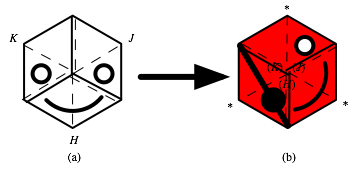FIGURE 7: The motion, flexing down

Beginning with the flexagon in the standard starting position, you may verify thatyields the same face as f 17 of FIGURE 6. Thus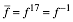. This means that, if you start with the position indicated on the right of FIGURE 7 and flex down, you get the initial position. In other words, flexing up is the inverse of flexing down (und vice versa), as you might expect.

If you're enjoying this you may check your flexing skill by reversing all of the steps of the Happy Group in FIGURE 6.

3. The Entire Group

We realize that the full group for this Hexagon must be larger than C18, because no frowning faces ever appeared under the motions f n. Cheerful as this situation is, it is plainly not complete. Like everything in this world this Hexagon has good (happy) and bad (unhappy) features. In order to get the entire group we certainly need to have a motion that makes the unhappy faces visible. To achieve this we introduce a new motion,

t = turn over (so the rotation is about a horizontal axis).

Thus, if we begin with the Hexagon in the standard initial position and perform the motion t we will see a yellow frowning face (see FIGURE 8).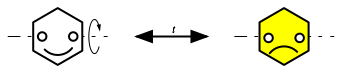FIGURE 8: The motion t, turning over

Obviously t is an involution, that is, t 2 = 1. FIGURE 9 shows that the motion f t (meaning first do t, then do f) is not the same as t f (meaning first do f, then do t). Check this (remembering that the flexagon should be in the standard initial position, in both cases, when you start). Thus we see that our new motion t does not commute with f.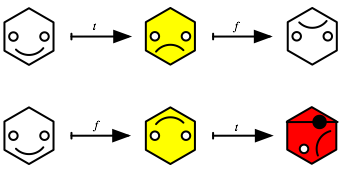FIGURE 9: f tt f

We also notice that when the pirate frowns his patch covers his left eye, instead of the right one!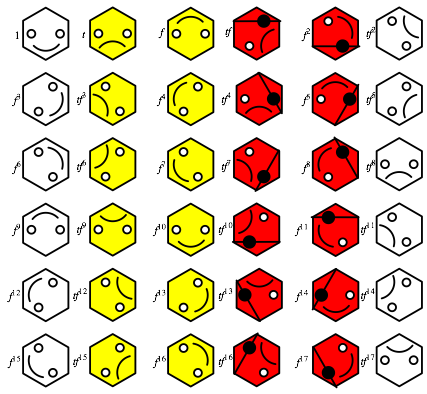FIGURE 10: The entire group

FIGURE 10 shows all the possibilities for f n and t f n. Notice that the first, third, and fifth columns are just the smiling faces from FIGURE 6. This observation may give you an idea of an easy way to confirm that the visages in FIGURE 10 are correct.

We have already seen that t f n f n t. However, since flexing up, as viewed from above the Hexagon, is the same as flexing down, as viewed from below the Hexagon, we have,

f n t = t f –n.

Thus we see that the group generated by f and t has 36 elements and is therefore the fuIl group of motions of our flexagon. Since the generators f, t satisfy the defining set of relations f 18 = 1, t 2 = 1, f t = t f –1, the group is the dihedral group D18, the group of symmetries of the regular 18-gon (shown in FIGURE 11). The figure on the front cover shows the effects of the group elements, where the single-headed arrows denote the f action and the double-headed arrows denote the t action.FIGURE 11: The regular 18-gon

4. A Normal Subgroup

If we are only interested in the different expressions on the faces of our Hexagon, without respect to orientation, we have only 6 cases (as seen in FIGURE 12), instead of 36.

FIGURE 10 motivates the following argument. We obtain the group of motions of the unoriented faces by adding the relation f 3 = 1 to our group D18. The resulting quotient group of D18 by the normal subgroup generated by f 3 is then generated itself by F and t, subject to F 3 = 1, t 2 = 1, F t = t F –1. Here, F is, of course, the image in the quotient group of f; and the quotient group is just the symmetric group S3.FIGURE 12: Expressions of the flexagon

5. A Challenge to the Reader

In  the tri-hexaflexagon was discussed and the group S3 was obtained by using a flexagon where each of the 3 faces simply had different colors. In  the group D9 was obtained by a systematic labeling of the vertices of the 6 triangles on each of the 3 faces of the tri-hexaflexagon. However, in order to obtain the entire group D18, it was necessary to introduce a finer method of distinguishing between the different orientations of the faces, distinguishing between smiling and frowning visages did the trick. The obvious next question to explore is whether or not this, or some refinement of it, will help to identify the mathematical structure of the hexa-hexa-flexagon (with 6 faces). If we had a quick, or easy, answer to this question we wouldn't be stopping here!

REFERENCES

1. Martin Gardner. The Scientific American Book of Mathematical Puzzles and Diversions. Simon and Schuster, New York, NY, 1959.

2. Michael Gilpin, Symmetries of the trihexaflexagon. Mathematics Magazine 49, No. 4 (1976), 189-192.

3. Peter Hilton and Jean Pedersen: Build Your Own Polyhedra. Addison-Wesley, Menlo Park, CA, 1994.

4. Jean Pedersen. Sneaking up on a group. Two-year College Mathematics Journal 3 (1972), 9-12.

 We may refer to the tri-hexaflexagon as simply “the flexagon” if no confusion would result.

 Notice that, from the point of view of decorating this piece, we have available a total of 20 triangles (because the strip of paper has two surfaces, the top surface and the bottom surface). When two of the triangles are glued to each other there remain 18 triangles with which to form the 3 faces.

 This is, of course, the same initial position as that in FIGURE 5(a), but the labeling has changed.

 Although we could do without any eye patches in analyzing this particular f1exagon, it is clear that this feature may provide a better way of keeping track of the faces on more complicated flexagons – and we thought the pirate made an interesting addition to this group (visually and socially!).